# 1st PUC Chemistry Previous Year Question Paper March 2013 (North)

Students can Download 1st PUC Chemistry Previous Year Question Paper March 2013 (North), Karnataka 1st PUC Chemistry Model Question Papers with Answers helps you to revise the complete Karnataka State Board Syllabus and score more marks in your examinations.

## 1st PUC Chemistry Previous Year Question Paper March 2013 (North)

Time: 3.15 Hours
Max Marks: 90

Instructions:

1. Write the question number legibly in the margin.
2. Answer for a question should be continuous.

Part – A

I. Answer the EIGHT of the following : ( 8 × 1 = 8 )

Question 1.
Write the IUPAC name of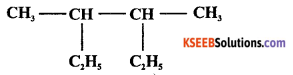3, 4- dimethyl hexane.

Question 2.
Draw the staggered conformation of ethane.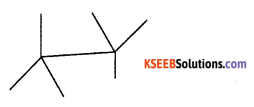Question 3.
State modern periodic law.
Properties of elements are periodic functions of their atomic numbers.

Question 4.
What is meant by octet rule?
It is the tendency of atom to attain 8 electrons in valence shell.Question 5.
Name the process in which hydrogen is obtained as a by product?
Electrolysis of brine solution.

Question 6.
Which alkali metal is the strongest reducing agent?
Lithium or Li.

Question 7.
How many significant figures in 6.022 × 1023?
4 or Four.

Question 8.
Which orbital is specified by l = 2 and n = 3?
3d.

Question 9.
State Boyle’s law.
At constant temperature the volume of a given mass of a gas is inversely proportional to the pressure.

Question 10.
Write relationship between ∆H and ∆U?
∆H = ∆U + RT∆n.Question 11.
What is effect of catalyst on the equilibrium of a reversible reaction.
A catalyst has no effect on the position of equilibrium but it helps the reaction to attain equilibrium quickly.

Part – B

II. Answer any EIGHT of the following questions. ( 8 × 2 = 16 )

Question 12.
Define functional group.
Atom or group of atom which determine the characteristic properties of organic compound.

Question 13.
State Markownikoff’s rule.
When an asymmetric reagent adds up to an unsymmetrical alkene, the negative part of the adding molecule goes to the carbon atom with lesser number of hydrogen atoms while the positive part to the other carbon atom.

Question 14.
What is electron gain enthalpy?
The enthalpy change occurs when an electron is added to isolated gaseous atom to convert into anion.

Question 15.
Write electronic configuration of C2 molecule ? What is its magnetic property?
$$\sigma_{1 \mathrm{s}^{2}} \sigma_{1 \mathrm{s}^{2}}^{*} \sigma_{2 \mathrm{s}^{2}} \sigma_{2 \mathrm{s}^{2}}^{*}\left(\pi 2 \mathrm{p}_{x}^{2}\right)\left(\pi 2 \mathrm{p}_{y}^{2}\right)$$ Diamagnetic.Question 16.
What is diagonal relationship? Give an example.
Lithium shows similarities to magnesium and beryllium to aluminium in many of their properties. This type of diagonal similarity is commonly referred to as diagonal relationship in periodic table.

Question 17.
Diamond is a bad conductor of electricity but graphite is a good conductor. Justify the statement.
Due to sp3 hybridisation in diamond, no free electrons are present.
In graphite due to sp2 hybridisation there are free electrons to conduct electricity.

Question 18.
What mass of calcium carbonate is to be decomposed to obtain 4.4 g of CO2 in the following reaction.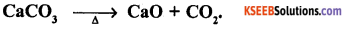(molecular mass of CaCO3 = 100g) .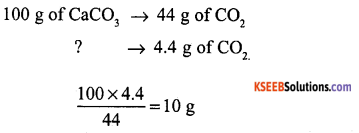Question 19.
State and illustrate Pauli’s exclusion principle.
No two electrons in an atom can have all same set of four quantum numbers alike.

Question 20.
Write Vander Waals equation for one mole of a gas and name any two terms.
$$\left(p+\frac{a}{V^{2}}\right)(V-b)=R T$$
P = pressure, V = volume, R = universal gas constant, T = absolute temperatureQuestion 21.
What type of system, the following systems are
i) a cup containing hot tea
ii) hot coffee placed in a thermos flask?
i) open system
ii) isolated system.

Question 22.
State Le Chatelier’s principle.
If a system under equilibrium be subjected to a change in temperature, pressure or concentratio, then the equilibrium shifts itself in such a way so as to neutralise the effect of the change.

Question 23.
Chemical equation is dynamic. Give reason.
Both forward and backward reactions are occuring at the same rate.
The concentration of the reactants and products remains constant.

Part – C

III. Answer any FOUR of the following questions : ( 4 × 4 = 16 )

Question 24.
What is resonance effect? Name one group each showing + R and – R effect.
The resonance effect is defined as the polarity produced in the molecule by the interaction of two π bonds or between a π -bond and lone pair of electrons present in conjugated molecular.
+Reffect : -NH2, – NHCOCH3, – CH3
-Reffect : -NO2, -CN, -CH0, -COOHQuestion 25.
Define chain and functional isomerism. Give examples for each isomerism.
Compounds having same molecular formula but differing in arrangement of atoms in chain is called isomerism.
Ex – Butane C4H10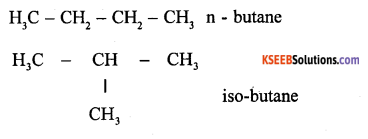Functional Isomerism
Compounds having same molecular formula but differing in functional group is called functional isomerism.
Ex :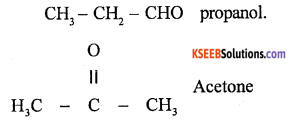Question 26.
(a) Explain the mechanism of nitration of benzene?
Nitration benzene reacts with a mixture of concentrated nitric acid and concentrated sulphuric acid at 50°C to form nitrobenzene.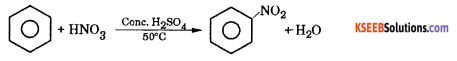Mechanism : This involves the following steps.
Step 1 : Generation of electrophile nitronium ion NO
HNO3 + 2H2SO4 → NO2++ H3O+ + 2HSO4

Step 2 : The electrophile NO2+ attacks the benzene ring to form a carbocation which is resonance stabilized.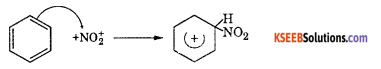Step 3 : Loss of a proton to give nitrobenzene. The proton is removed by HSO4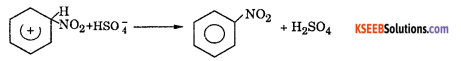(b) Mention the catalyst used in Friedel Crafts reaction.
anhydrous AlCl3.Question 27.
(a) Explain the mechanism of chlorination of methane.
Mechanism of chlorination of methane involves three types.
Step 1 : Initiation : Chlorine absorbs energy and undergoes homolysis to give chlorine free radicals.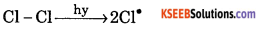Step 2 : Propagation : Chlorine free radical reacts with methane to give methyl free radical.
Cl+ CH4 → CH3+HCl

The methyl free radical reacts with chlorine to form methyl chloride and chlorine free radical.
CH3+ Cl2 → CH3Cl + Cl

Step 3 : Termination : Free radiais combine to form stable products.
Cl + Cl → Cl (Chlorine)
CH3+CH3→ C2H6 (Ethane)
CH3+ Cl → CH3Cl (Methyl Chloride)

(b) Draw the structure of trans 1, 2- dibromo ethene.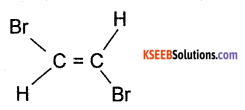Question 28.
(a) Define geometrical isomerism. Give an example.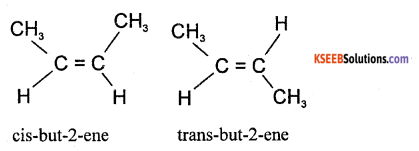restricted or hindered rotation about C = C bond is called geometrical isomerism.

(b) Name the product when calcium carbonte is treated with water.
Acetylene.Question 29.
What is pollutant? Mention the three gaseous air pollutants.
Chemicals which bring about undesirable changes in the environment are called pollutants.
Ex : Nitric oxide (NO), Nitrogen dioxide (NO2), sulphur dioxide (SO2)

Part – D

Answer any FIVE of the following questions : ( 5 × 5 = 25 )

Question 30.
(a) Define ionisation enthalphy. How does it vary along the period and down the group in the periodic table.
The minimum amount of energy which is needed to remove the most loosely bounded electron from a neutral isolated gaseous atom in its ground state to form a cation also in a gaseous state.

The ionisation enthalpy decreases down the group because the atomic size increases down the group and effective nuclear charge decreases. Hence removal of electrons become easier.

Ionisation energy increases along the period because the atomic size decreases from left to right in a period. Hence effective nuclear charge increases and removal of electrons becomes more and more difficult.

(b) How many periods are there in the periodic table?
7 – periods.

Question 31.
(a) Draw the energy level diagram of O2 molecule and calculate the bond order, why O2 is paramagnetic?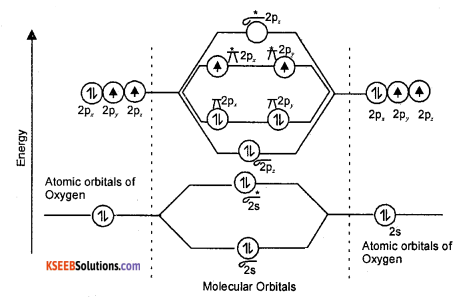Energy level diagram of oxygen molecule
Bond order = $$\frac{N_{b}-N_{a}}{2}=\frac{8-4}{2}=\frac{4}{2}=2$$

(b) Give two differences between bonding and antibonding molecular orbitals.

 Bonding molecular orbitals Anti-bonding molecular orbitals 1. Formed by the addition wave functions atomic orbitals. 1. Formed by the subtraction of wave functions atomic orbitals. 2. Have less energy than the atomic orbitals which combined 2. Have more energy than the atomic orbitals which combined.

Question 32.
(a) What is hydrogen bond? Give an example each for inter and intra molecular hydrogen bond.
A hydrogen bond is the attractive force which binds hydrogen atom of one molecule with electronegative atom CF1 O or N present in another molecule.
Intermolecular hydrogen bond – Ex -HF
Intramolecular hydrogen bond – Ex – O – nitrophenol.

(b) According to VSEPR theory, predict the shape of H2O and NH3 molecules.
H2O – bent V shaped.
NH3 – pyramidal.

Question 33.
(a) Balance the following redox reaction using oxidation number method.
Cr2O7-2+ S2- + H+ → Cr3+ + S + H2O.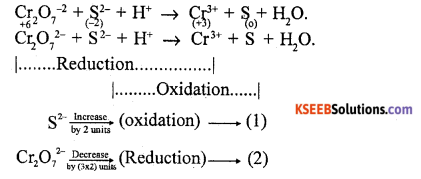Multiply oxidation equation by the extent of reduction & reduction equation by the extent of oxidation.
Eqn (1) × 6; 6S2- → 6S
Eqn (2) × 2; 2Cr2O72 → 4Cr3+
2CrO-+ 6S2- → 4Cr3+ + 6S
Balance oxygen
2CrO 2-+ 6S2- → 4Cr3++ 6S + 14 H2O
Balance hydrogen
2Cr2O72-+ 6S2- + 28H+ → 4Cr3++ 6S + 14 H2O

(b) Identify the type of redox reactions.
i) Zn (s) + CuSO4 (aq) → ZnSO4 (aq) + Cu(s).
ii)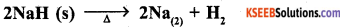i) Displacement type
ii) Decomposition typeQuestion 34.
(a) What happens when metal Mg is treated with dilute hydrochloric acid and write the equation.
Mg + 2HCl → MgCl2 + H2
When Mg is treated with HCl, MgCl2 and H displaces hydrogen gas.

(b) Why are metallic hydrides also called interstitial hydrides.
In these hydrides, hydrogen occupies interstices in the metal lattice producing distortion without any change in its type so they are also called interstitial hydrides.

(c) Give two uses of hydrogen.

1. Dihydrogen is used in the manufacture of vanaspathi fat by the hydrogenation of polyunsaturated vegetable oil.
2. Widely used in the manufacture of metal hydrides.

Question 35.
(a) Name the raw materials used in manufacture of Na2CO3 by Solvay process.
Ammonia, CO2, sodium chloride, ammonium hydrogen carbonate.

(b) Mention biological role of Na and Ca ions.
Na – are involved in transmission of nerve signals.
Ca – 99% of body calcium is present in bones and teeth.

(c) Which alkali metal gives golden yellow colour to the flame?
Sodium.

Question 36.
(a) Define catenation and name the element showing maximum property of catenation.
Self linking of carbon atom to form a long /branched chain/cyclic ring is called catenation.
Carbon has maximum catenation property.

(b) What is the repeating unit in Organo Silicon polymer? Name the starting material used in the manufacture of Organo Silicon Polymer.
(-R2SiO-) is the repeating unit.
Methyl chloride and silicon in presence of copper as catalyst at temperature 573 K are the starting material.

OR (Internal Choice)Question 36.
(a) Name the compound of group – 13 element which exist as dimer and draw the structure of the compound.
AlCl3 Structure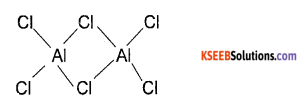(b) What is inert pair effect? Illustrate it with suitable example.
The occurrence of oxidation states two unit less than that of group oxidation states are attributed as inert pair effect.
Ex : Ge, Sn and Pb prefer to exhibit + 2 state because of inert pair effect i.e. ns2 electrons remain inert and do not involve in bonding.

Part – E

Answer any FIVE of the following questions :  ( 5 × 5 = 25 )

Question 37.
(a) carbohydrate containing 40% carbon, 6.73% hydrogen and 53.3% oxygen. The molecular mass of compound is 180. Determine its molecular formula.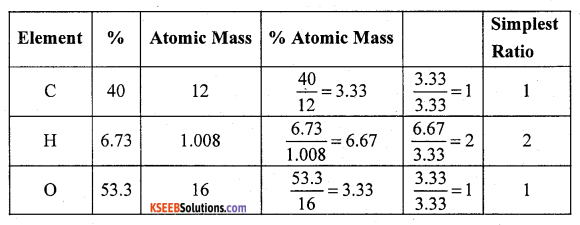∴ Empirical formula = CH2O.
Molecular mass of compound =180
Empirical mass = CH2O. = 12 + 2 × 1.008 + 16 = 30
$$\mathrm{n}=\frac{\mathrm{M} \cdot \mathrm{M}}{\mathrm{E} \cdot \mathrm{M}}=\frac{180}{30}=6$$
Molecular formula = C6H12O6 = Glucose

(b) Define empirical formula.
It is the simplest formula of compound which gives the relative number of atoms of different elements present in a molecule of a compound.Question 38.
(a) Calculate the wavelength of spectral line when an electron in hydrogen atom undergoes transition from 3rd energy level to 2nd energy level (R = 109678 cm-1).
$$\frac{1}{\lambda}$$ = R $$\left[\frac{1}{n_{1}^{2}}-\frac{1}{n_{2}^{2}}\right]$$ = 109678 cm-1 $$\left[\frac{1}{2^{2}}-\frac{1}{3^{2}}\right]$$
$$\frac{1}{\lambda}$$ = 15245.242 cm-1
l = $$\frac{1}{15245.242 \mathrm{cm}^{-1}}$$ = 0.00006559 cm = 6.559 × 1O-5 cm.

(b) Mention the shape of d-orbitals.
double dumbell shaped.

Question 39.
(a) Write four postulates of kinetic theory of gases.

1. All gases are made up of a large number of very small particles called molecules.
2. The gas molecules move randomly in all possible directions in straight lines. The direction of motion changes when they collide with other molecules.
3. The molecules are so small that their individual values is negligible as compared to the total volume of gas.
4. There is no force of attraction or repulsion between the molecules of a gas.

(b) What is the value of gas constant.
R = 8.314 JK-1 mol-1.

Question 40.
(a) Calculate the enthalpy of formation of liquid benzene (C6H6) given the enthalpies of combustion of carbon, hydrogen and benzene as -393.5 KJ, -285.83 KJ and -3267.0 KJ respectively.
Required equation
6C(s) + 3H2(g) → C6H6 (l) ΔfH = ?
Given Data : C(s) + O2(g) → CO2(g)  ΔcH = -393.5KJ
H2(g) + $$\frac{1}{2}$$ O2(g) → H2O(l)  ∆cH = -285.83K
C6H6(l) + $$\frac{15}{2}$$ O2(g) → 6CO2 + 3H2O  ΔcH = -3267. 0KJ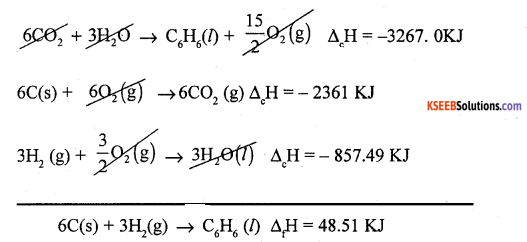(b) What is aspontaneous process?
A spontaneous process is one which occurs by itself without any external aid.

OR (Internal choice)

(a) Calculate the enthalpy of combustion of methanol (CH3OH) given enthalpies of formation of CH3OH (1) CO2(g) and H2O(1) as -239 KJ, -393.5 KJ and -286 KJ respectively.
Required Eqn. CH3OH(l) + $$\frac{3}{2}$$O2(g) → f CO2(g) + 2H2O(l)  ∆cH = -?
Given data
(1) C(s) + 2H2(g) + $$\frac{1}{2}$$ O2(g) → CH3OH(l)  ∆fH = = -239 kJ
(2) C(s) + O2(g) → CO2(g)   ∆fH = -393.5 Id
(3) H2(g) + $$\frac{1}{2}$$O(g) → H2O(l)  ∆fH = -286 kJ
Reverse eqn( 1) and multiply 3 by 2 and add all three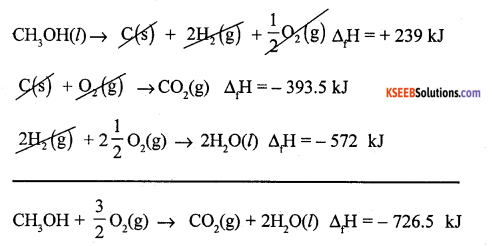(b) What happens to the entropy, when liquid is converted into vapours?
Entropy increases.Question 41.
(a) Calculate K. for the following equilibrium 2SO2(g) + O2(g) 2SO3(g). Given equilibrium constant of SO2,O2 and SO3 are 0.3 M, 0.41 M, and 1.45 M respectively. What happens to the above equilibirum if (i) SO2 is added (ii) O2 is removed.
$$K_{c}=\frac{\left[\mathrm{SO}_{3}\right]^{2}}{\left[\mathrm{SO}_{2}\right]^{2}\left[\mathrm{O}_{2}\right]}$$
= $$\frac{(1.45)^{2}}{(0.3)^{2}(0.41)}$$ = 56.97
i) Addition of SO2 favours forward reaction.
ii) Removal of O2 favours backward reaction.

(b) Identify the two conjugate acid base pairs in the following NH3 + HCl NH4++ Cl
NH3 / NH4+ , HCl / Cl

Question 42.
(a) What is common ion effect? Give an example.
Suspension of degree of dissociation of weak electrolyte by the addition of strong electrolyte having a common ion is called common ion effect.

(b) Calculate the solubility of lead chloride (PbCl2) at 298K, if its solubility product is 1.6 × 10-5.
$$S=\sqrt{\frac{K_{s p}}{4}}=\sqrt{\frac{1.6 \times 10^{-5}}{4}}=1.58 \times 10^{-2} \mathrm{mol} / \mathrm{dm}^{3}$$

Question 43.
(a) State postulates of Bohr’s theory.

1. Electrons revolve around the nucleus in an circular path of fixed radius and energy. These paths are called orbits.
2. The energy of an electron in the orbit does not change with time.
3. The frequency of radiation absorbed or emitted when transition occurs between two stationary states that differ in energy by ∆E.
$$\gamma=\frac{\Delta \mathrm{E}}{\mathrm{h}}=\frac{\mathrm{E}_{2}-\mathrm{E}_{1}}{\mathrm{h}}$$
4. The angular momentum of an electron in a given stationary state can be expressed as
mvr = n. $$\frac{h}{2 \pi}$$ n = 1, 2, 3……

(b) Write de Broglies equation.
λ = $$\frac{\mathrm{h}}{\mathrm{mv}}$$ or $$\frac{\mathrm{h}}{\mathrm{mc}}$$Question 44.
(a) Define the terms molarity and mole fraction.
Molarity (M) = $$\frac{\text { No. of moles of solute }}{\text { Volume of solution in litres }}$$
Mole fraction of A = $$\frac{\text { No. of moles of } \mathrm{A}}{\text { No. of moles of solution }}$$
= $$\frac{\mathbf{n}_{A}}{n_{A}+n_{B}}$$